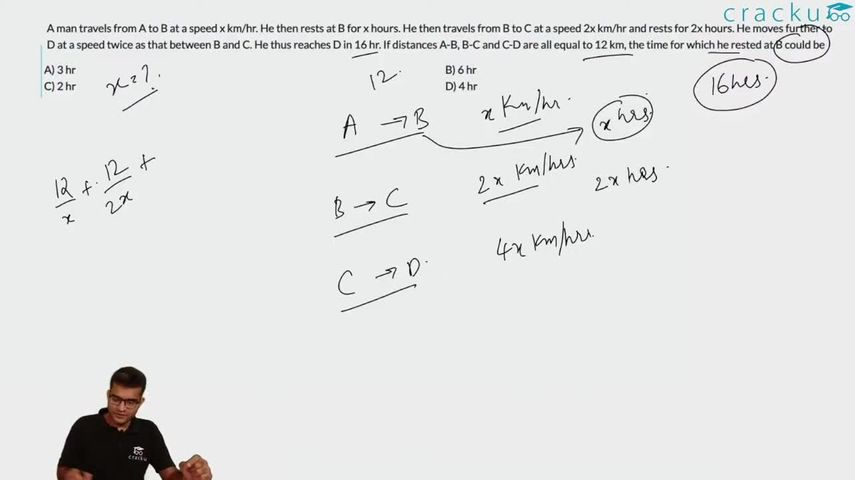Question 34

# A man travels from A to B at a speed x km/hr. He then rests at B for x hours. He then travels from B to C at a speed 2x km/hr and rests for 2x hours. He moves further to D at a speed twice as that between B and C. He thus reaches D in 16 hr. If distances A-B, B-C and C-D are all equal to 12 km, the time for which he rested at B could be

Solution

Total time taken to reach at D:

$$\frac{12}{x} + x + \frac{12}{2x} + 2x + \frac{12}{4x} = 16$$

Or $$3x^2 - 16x + 21 = 0$$

From the options we can see that only, $$x$$ = 3hr satisfies the equation. Thus, A is the right choice.

### View Video Solution